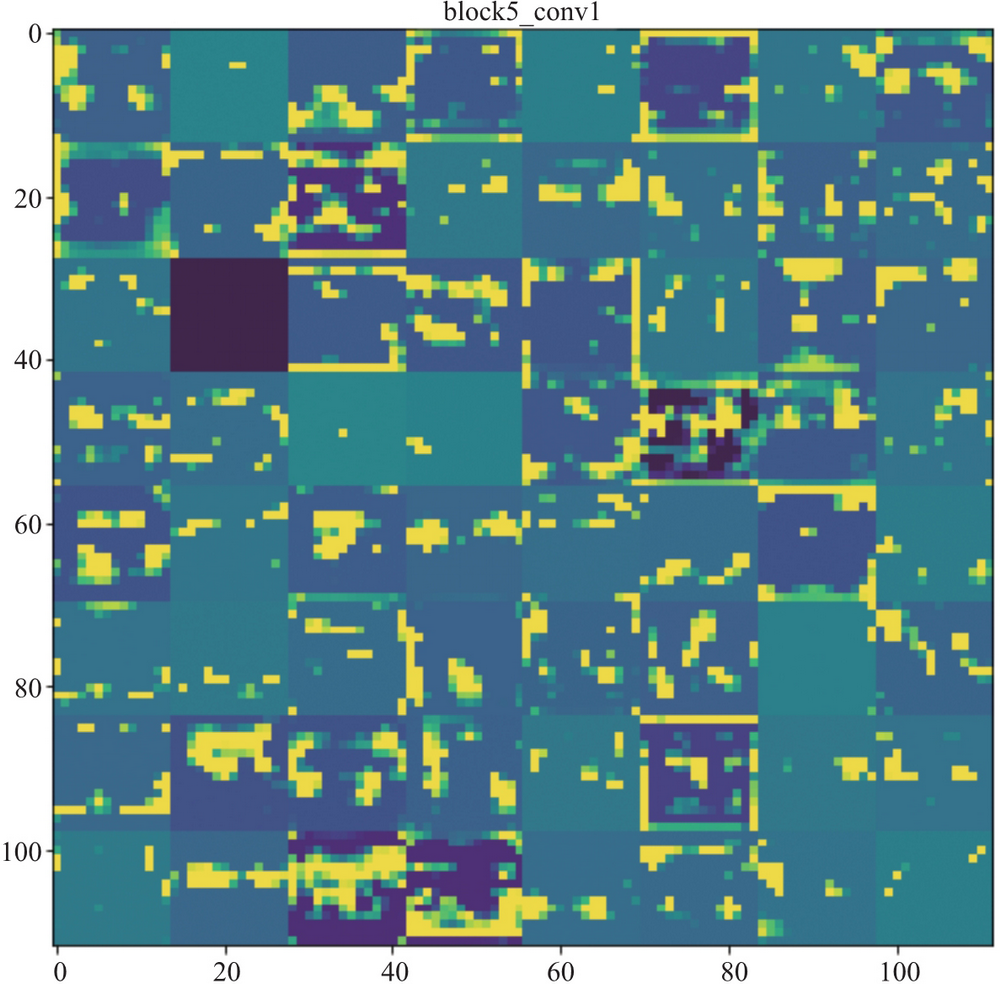# AI 安全之对抗样本入门 (13)：深度学习基础知识 1.3.9(可视化 CNN)

CNN 虽然接近人类识别物体的过程，但是理解 CNN 的原理却是非常艰难的过程。人们试图用可视化的方式来理解 CNN 各层对图像特征提取的方法。Keras 之父、谷歌大脑人工智能和深度学习研究员 Francois Chollet 在他的《Python 深度学习》1中给出一种可视化卷积的方法。卷积层通常由多个卷积核组成，每个卷积核都可以被视为一种特征提取方式。当使用一个卷积核处理图像数据后，卷积核会提取它关注的特征并形成新的图像，该图像也被称为特征图。输入的图像包含的特征与卷积核越接近，其特征图的值也越大。因此完全可以基于梯度，迭代调整输入图像的值，让特征图的值最大化。当特征图的值达到最大或者迭代求解趋于稳定时，可以认为这时的输入图像就是该卷积核的可视化图像。下面以 Keras 为例介绍核心代码实现。

1 图片源于 http://blog.csdn.net/numeria/article/details/73611456

#获取输出层的 tensorlayer_output = model.get_layer(layer_name).output#获取指定卷积核 filter_index 的输出，并作为损失函数loss = K.mean(layer_output[:, :, :, filter_index])#根据损失函数和输入层定义梯度grads = K.gradients(loss, model.input)grads /= (K.sqrt(K.mean(K.square(grads))) + 1e-5)#实例化计算梯度的函数iterate = K.function([model.input], [loss, grads])#定义一个随机图像，图像底色为灰色，并叠加均值为 0 标准差为 20 的高斯噪声input_img_data = np.random.random((1, size, size, 3)) * 20 + 128.#迭代 40 轮，使用梯度上升算法求解，学习速率（步长）为 stepstep = 1.for i in range(40):        loss_value, grads_value = iterate([input_img_data])        input_img_data += grads_value * stepimg = input_img_data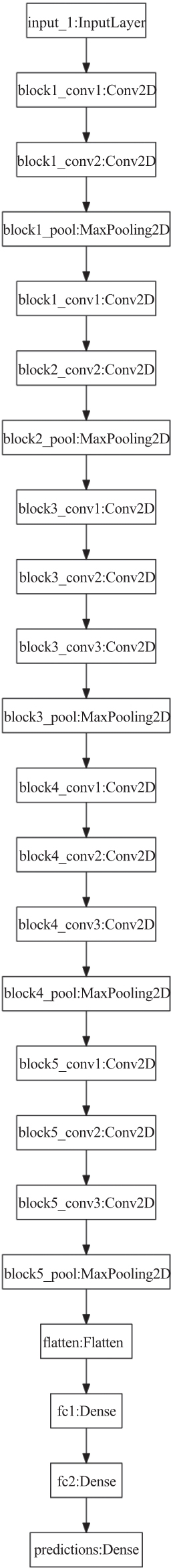Francois Chollet 使用上述方法可视化了这 5 个卷积层，block1_conv1 的可视化结果如图 1-25 所示，block3_conv1 的可视化结果如图 1-26 所示，block5_conv1 的可视化结果如图 1-27 所示，可见第 1 层卷积提取的主要是边缘和纹路特征，越往后的卷积提取的特征越高级，到了第 5 层卷积已经提取很抽象的高级特征了。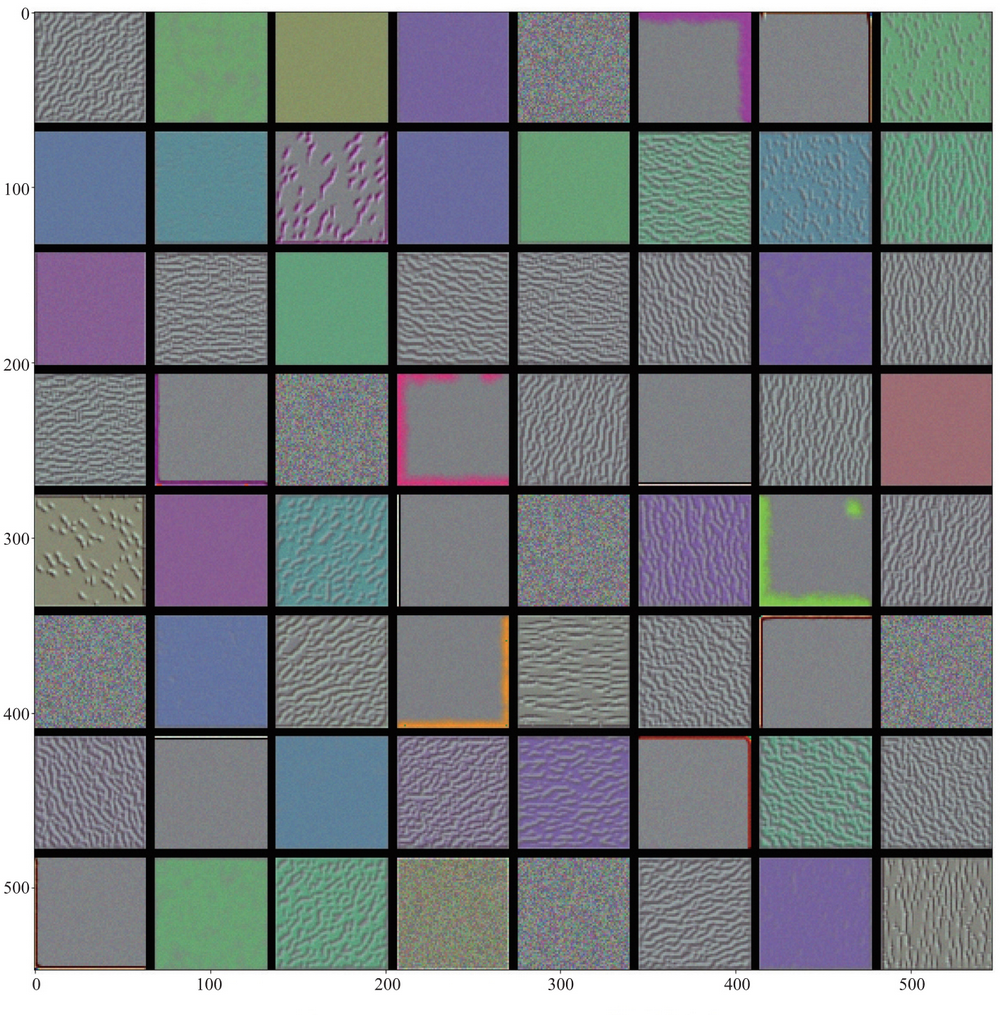https://github.com/duoergun0729/adversarial_examples/code/1-case2-keras.ipynb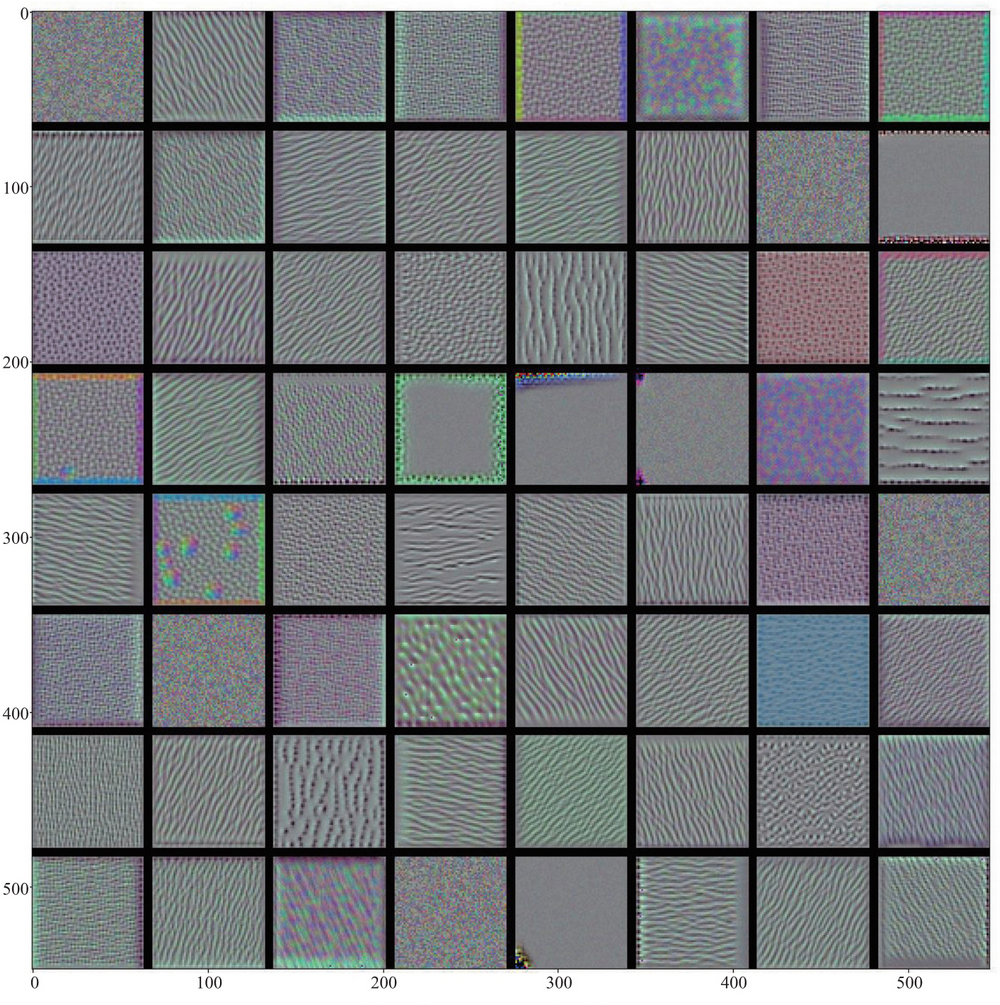#使用 VGG16from keras.applications.vgg16 import VGG16import matplotlib.pyplot as plt%matplotlib inlinemodel = VGG16(weights='imagenet')

from keras.preprocessing import imagefrom keras.applications.vgg16 import preprocess_input, decode_predictionsimport numpy as np#小猪的路径img_path = "../picture/pig.jpg"#缩放到指定大小 224×224img = image.load_img(img_path, target_size=(224, 224))#展示图片plt.imshow(img)plt.show()x = image.img_to_array(img)# 扩展维度，适配模型输入大小 (1, 224, 224, 3)x = np.expand_dims(x, axis=0)# 图像预处理x = preprocess_input(x)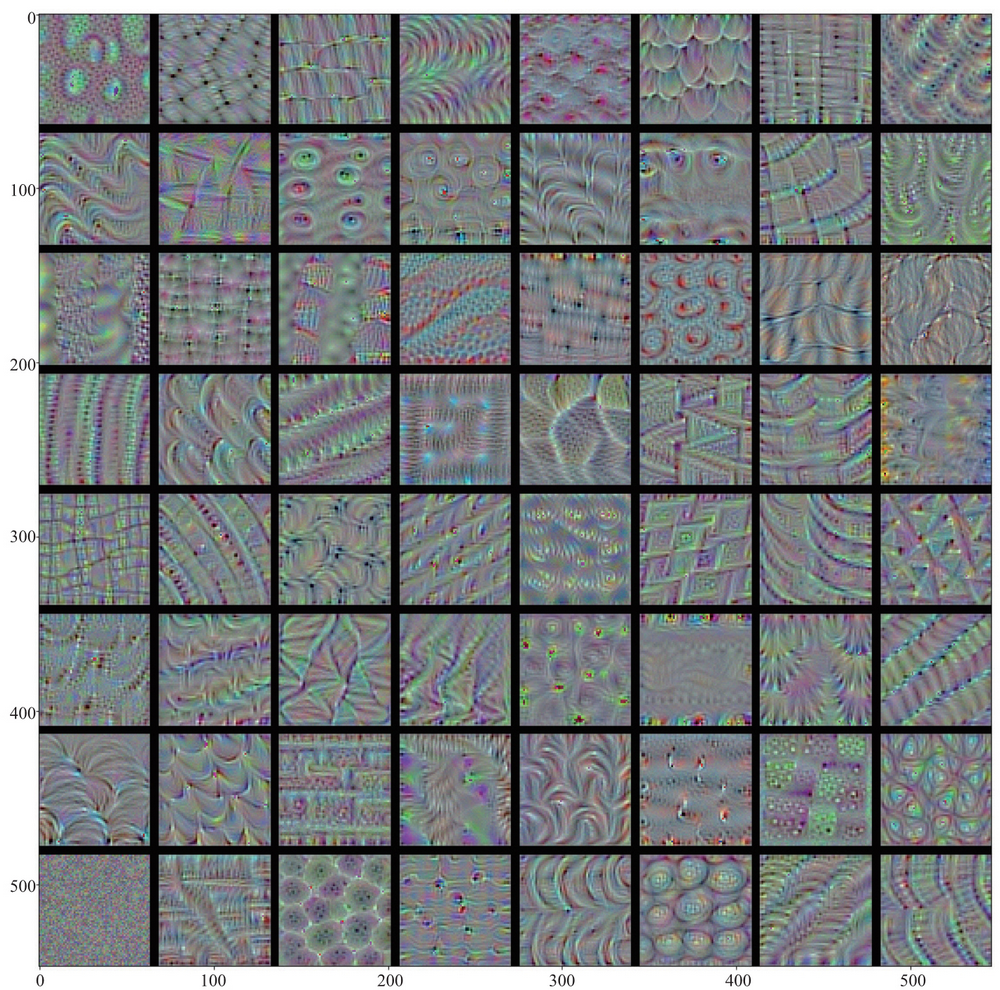1 图 1-25 至图 1-27 源于 https://github.com/fchollet/deep-learning-with-python-noteboos/blob/master/5.4-visualizing-what-convnets-learn.ipynb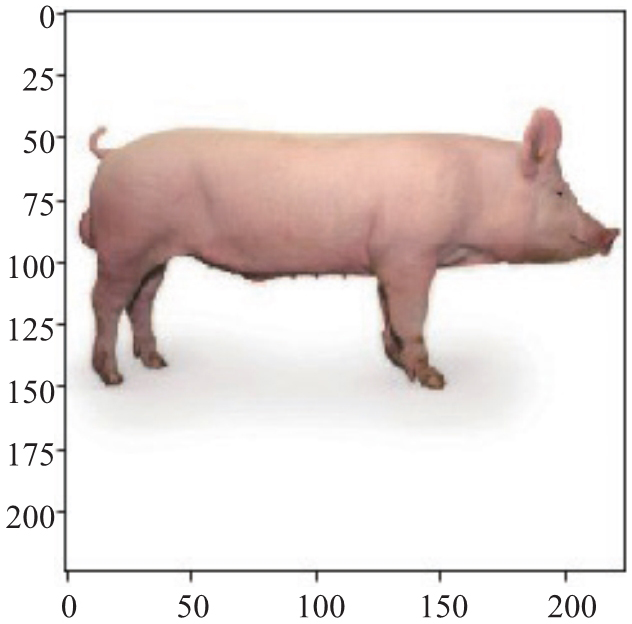preds = model.predict(x)print('Predicted:', decode_predictions(preds, top=3))Predicted: [('n03935335', 'piggy_bank', 0.6222573), ('n02395406', 'hog',0.3228228), ('n02108915', 'French_bulldog', 0.013370045)]

from keras import modelslayer_names=['block1_conv1', 'block3_conv1','block5_conv1']# 获取指定层的输出:layer_outputs = [model.get_layer(layer_name).output for layer_name inlayer_names]# 创建新的模型，该模型的输出为指定的层的输出activation_model = models.Model(inputs=model.input, outputs=layer_outputs)

#获得小猪的输出activations = activation_model.predict(x)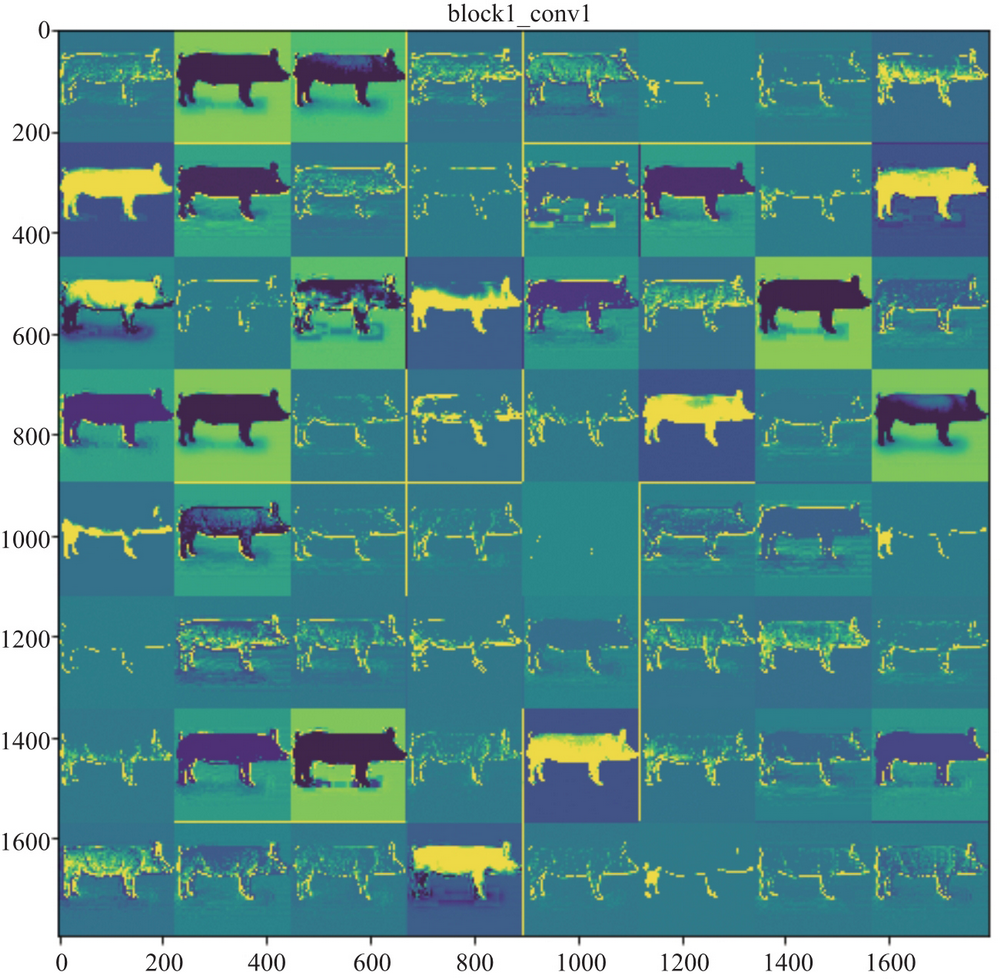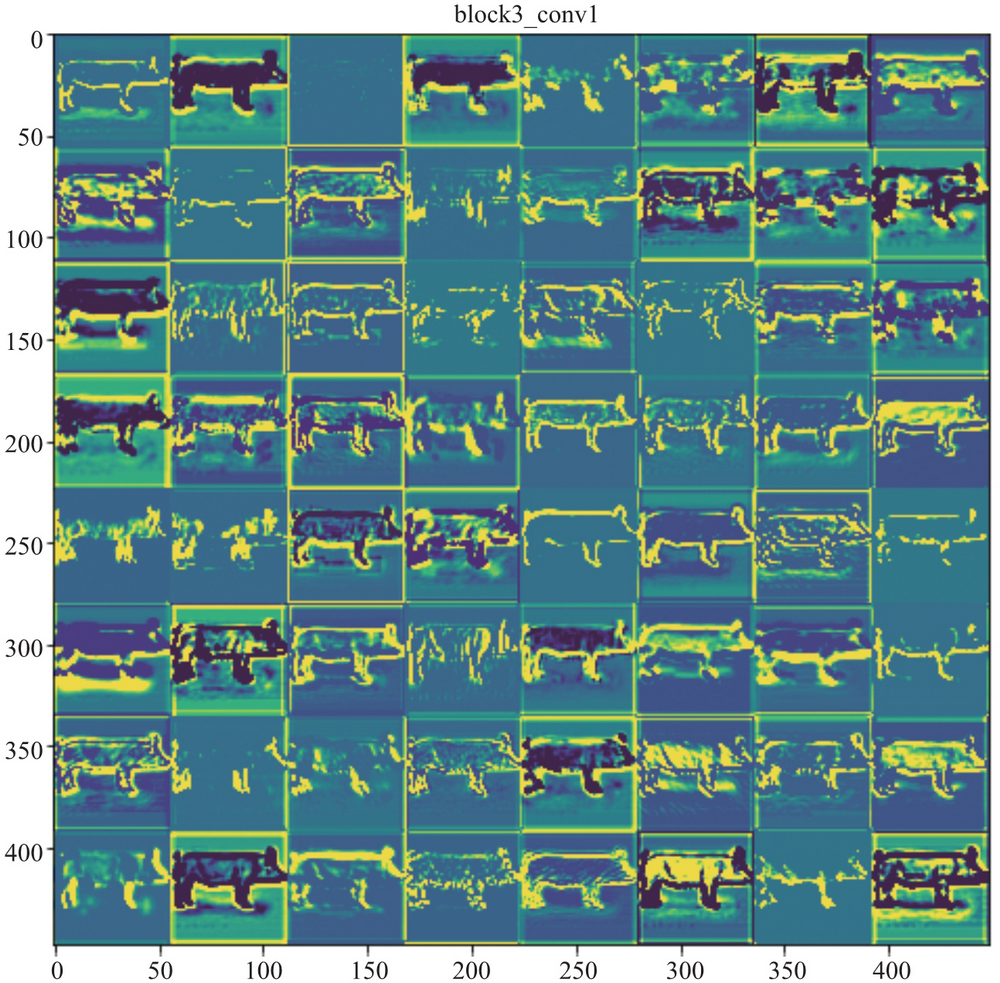images_per_row = 8for layer_name, layer_activation in zip(layer_names, activations):    # 获取卷积核的个数    n_features = layer_activation.shape[-1]    # 特征图的形状 (1, size, size, n_features)    size = layer_activation.shape    #最多展现 8 行    n_cols=8    display_grid = np.zeros((size * n_cols, images_per_row * size))    for col in range(n_cols):        for row in range(images_per_row):            channel_image = layer_activation[0,:, :,                                               col * images_per_row + row]            # 归一化处理            channel_image -= channel_image.mean()            channel_image /= channel_image.std()            # 数据扩展到 [0,255] 范围            channel_image *= 128            channel_image += 128            channel_image = np.clip(channel_image, 0, 255).astype('uint8')            display_grid[col * size : (col + 1) * size,                         row * size : (row + 1) * size] = channel_image    # 展示图片    scale = 1. / size    plt.figure(figsize=(scale * display_grid.shape,                        scale * display_grid.shape))    plt.title(layer_name)    plt.grid(False)    plt.imshow(display_grid, aspect='auto', cmap='viridis')plt.show()